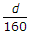# Civil Engineering - UPSC Civil Service Exam Questions

21.

The percentage of time in a year during which the cross wind component remains within the limit, is :

 A. Wind coverage B. Headwind C. Prevailing wind D. Cross wind

Explanation:

No answer description available for this question. Let us discuss.

22.

Consider the following statements :
A concrete mixer is specified by the :
1. volume of the mixing drum.
2. horse power of the prime mover.
3. volume of mixed concrete discharged.
4. mixer drum speed.
5. feeding arrangement.
Of these statements :

 A. 1, 2 and 5 are correct B. 1, 3 and 4 are correct C. 3 and 5 are correct D. 2 and 4 are correct

Explanation:

No answer description available for this question. Let us discuss.

23.

Choose the correct statement about horizontal component of resultant hydrostatic pressure on a curved submerged surface :

 A. It is equal to the product of pressure at the centroid and the curved area B. It is equal to the weight of the liquid above the curved surface acting at 0.5 depth of the surface C. It is equal to the projected area of the surface on a vertical plane multiplied by the pressure at the centre of gravity of area D. It is equal to the weight of the liquid above the curved surface multiplied by the projected area on a vertical plane.

Explanation:

No answer description available for this question. Let us discuss.

24.

A circular area of radius 'R' on the surface of a semi-infinite soil mass is uniformly loaded with a loading intensity of 'q'. The vertical stress σz directly below its centre at a depth 'z', is given by

 A.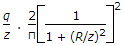B.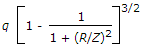C.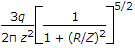D.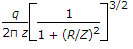Explanation:

No answer description available for this question. Let us discuss.

25.

The thickness of web for unstiffened plate girder with clear distance d between the flanges shall not be less than

 A.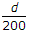B.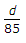C.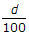D.